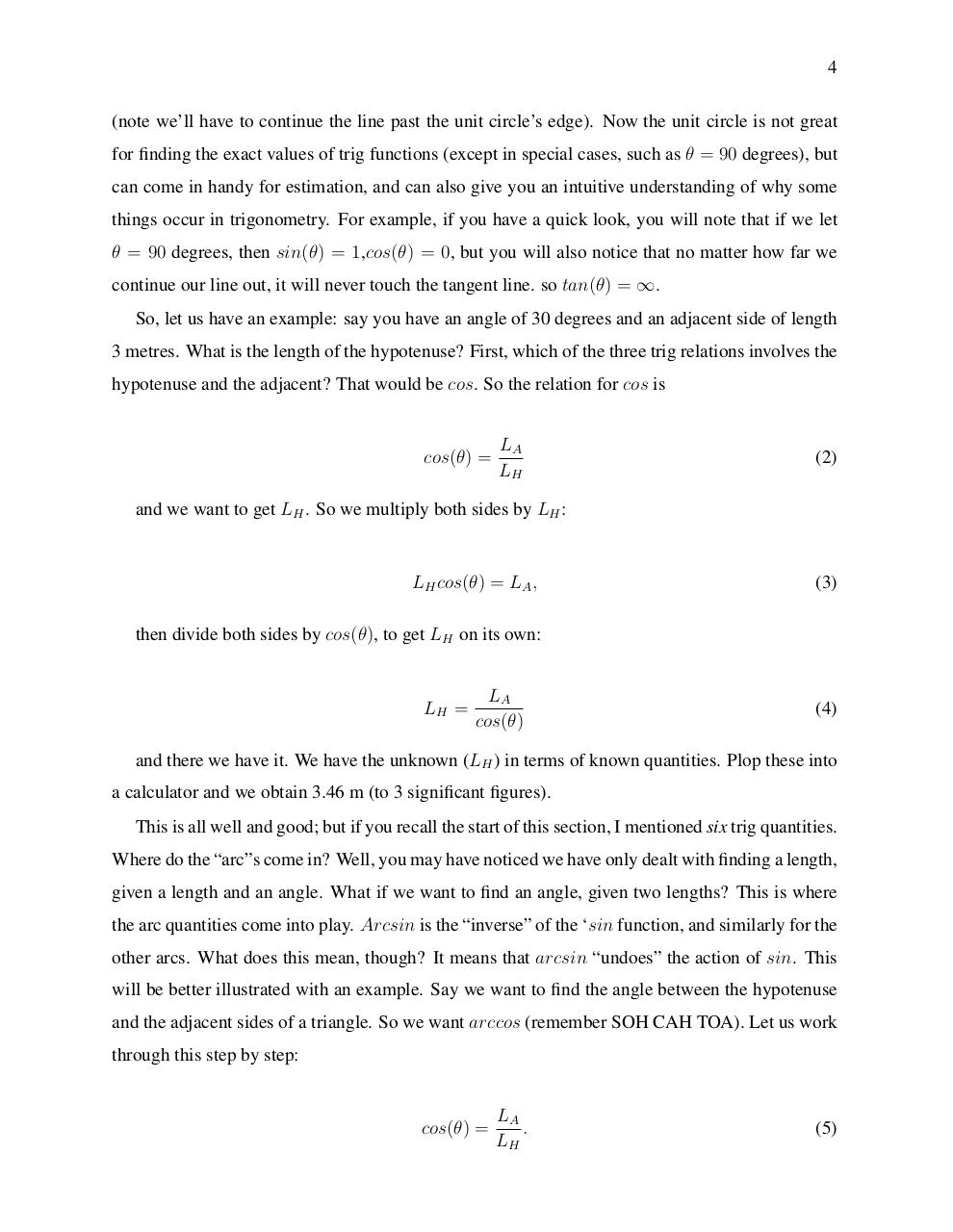# GDMathsGuide.pdfPage 1 2 3 45615

#### Text preview

4
(note we’ll have to continue the line past the unit circle’s edge). Now the unit circle is not great
for finding the exact values of trig functions (except in special cases, such as θ = 90 degrees), but
can come in handy for estimation, and can also give you an intuitive understanding of why some
things occur in trigonometry. For example, if you have a quick look, you will note that if we let
θ = 90 degrees, then sin(θ) = 1,cos(θ) = 0, but you will also notice that no matter how far we
continue our line out, it will never touch the tangent line. so tan(θ) = ∞.
So, let us have an example: say you have an angle of 30 degrees and an adjacent side of length
3 metres. What is the length of the hypotenuse? First, which of the three trig relations involves the
hypotenuse and the adjacent? That would be cos. So the relation for cos is

cos(θ) =

LA
LH

(2)

and we want to get LH . So we multiply both sides by LH :

LH cos(θ) = LA ,

(3)

then divide both sides by cos(θ), to get LH on its own:

LH =

LA
cos(θ)

(4)

and there we have it. We have the unknown (LH ) in terms of known quantities. Plop these into
a calculator and we obtain 3.46 m (to 3 significant figures).
This is all well and good; but if you recall the start of this section, I mentioned six trig quantities.
Where do the “arc”s come in? Well, you may have noticed we have only dealt with finding a length,
given a length and an angle. What if we want to find an angle, given two lengths? This is where
the arc quantities come into play. Arcsin is the “inverse” of the ‘sin function, and similarly for the
other arcs. What does this mean, though? It means that arcsin “undoes” the action of sin. This
will be better illustrated with an example. Say we want to find the angle between the hypotenuse
and the adjacent sides of a triangle. So we want arccos (remember SOH CAH TOA). Let us work
through this step by step:

cos(θ) =

LA
.
LH

(5)